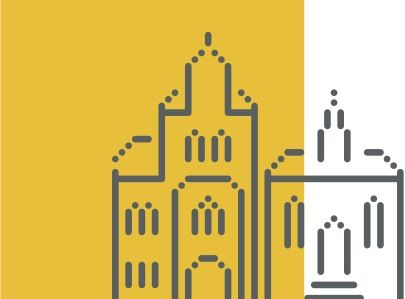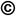TOP
##### Search the Dagstuhl Website
Looking for information on the websites of the individual seminars? - Then please:
Not found what you are looking for? - Some of our services have separate websites, each with its own search option. Please check the following list:##### Seminars
###### External resources:
• DOOR (for registering your stay at Dagstuhl)
• DOSA (for proposing future Dagstuhl Seminars or Dagstuhl Perspectives Workshops)
##### dblp
###### External resources:
• the dblp Computer Science Bibliography

### Beyond-Planar Graphs: Combinatorics, Models and Algorithms

##### Organizers
• (The University of Sydney, AU)
• (Universität Tübingen, DE)
• (EPFL - Lausanne, CH)
• (California State University - Northridge, US)

##### Motivation

Most big data sets are relational, containing a set of objects and relations between the objects. This is commonly modeled by graphs, with the objects as the vertices and the relations as the edges. A great deal is known about the structure and properties of special types of graphs, in particular planar graphs. The class of planar graphs is fundamental for both Graph Theory and Graph Algorithms, and has been extensively studied. Many structural properties of planar graphs are known, for example, in terms of excluded minors, low density, and small separators. These properties lead to efficient algorithms; consequently a number of fundamental algorithms for planar graphs have been discovered.

However, most real world graphs, such as social networks and biological networks, are nonplanar. In particular, the class of scale-free networks, which can be used to model web-graphs, social networks and many kinds of biological networks, are sparse nonplanar graphs, with globally sparse and locally dense structure. To analyze and visualize such real world networks, we need to solve fundamental mathematical and algorithmic research questions on sparse nonplanar graphs. Sparsity, in most cases, is explained by properties that generalize planar graphs: in terms of topological obstructions or forbidden intersection patterns among the edges.

A recent research topic in topological graph theory generalizes the notion of planarity to beyond-planar graphs, (i.e., nonplanar graphs that admit a planar representation that follows certain topological constraints such as specific types of crossings, or avoiding some forbidden crossing patterns), including:

• k-planar graphs: graphs that can be drawn with at most k crossings per edge;
• k-quasi-planar graphs: graphs which can be drawn without k mutually crossing edges;
• k-gap-planar graphs: graphs that admit a drawing in which each crossing is assigned to one of the two involved edges and each edge is assigned at most k of its crossings;
• RAC (Right Angle Crossing) graphs: graphs that have straight-line drawings in which any two crossing edges meet in a right angle;
• bar k-visibility graphs: graphs whose vertices are represented as horizontal segments (bars) and edges are represented as vertical lines connecting bars, intersecting at most k bars;
• fan-crossing-free graphs: graphs which can be drawn without fan-crossings; and
• fan-planar graphs: graphs which can be drawn such that every edge is crossed only by pairwise adjacent edges (fans).

This Dagstuhl Seminar will investigate beyond-planar graphs. It will explore, in particular, their combinatorial structure (i.e., thickness, density, coloring), geometric and topological obstructions (i.e., crossing numbers, stretchability, polyline drawing, geometric representation), recognition algorithms and applications (optimization, real-world network visualization). Behind the fundamental scientific challenges of this seminar lies the pragmatic need for better network visualization algorithms.Seok-Hee Hong, Michael Kaufmann, János Pach, and Csaba D. Tóth

##### Summary

Most big data sets are relational, containing a set of objects and relations between the objects. This is commonly modeled by graphs, with the objects as the vertices and the relations as the edges. A great deal is known about the structure and properties of special types of graphs, in particular planar graphs which are fundamental for both Graph Theory, Graph Algorithms and Automatic Layout. Structural properties of planar graphs can often be expressed, for example, in terms of excluded minors, low density, and small separators. These properties lead to efficient algorithms; consequently a number of fundamental algorithms for planar graphs have been discovered. As many of the characteristic properties of planar graphs have been generalized (e.g., graph minor theory, topological obstructions, chi-boundedness), these algorithms also extend in various directions to broad families of graphs.

Typical real world graphs, such as social networks and biological networks, are nonplanar. In particular, the class of scale-free networks, which can be used to model web-graphs, social networks and many kinds of biological networks, are sparse nonplanar graphs, with globally sparse and locally dense structure. To analyze and visualize such real world networks, we need to formulate and solve fundamental mathematical and algorithmic research questions on sparse nonplanar graphs. Sparsity, in most cases, is explained by properties that generalize those of planar graphs: in terms of topological obstructions or forbidden intersection patterns among the edges. These are called beyond-planar graphs. Important beyond-planar graph classes include the following:

• k-planar graphs: graphs that can be drawn with at most k crossings per edge;
• k-quasi-planar graphs: graphs which can be drawn without k mutually crossing edges;
• k-gap-planar graphs: graphs that admit a drawing in which each crossing is assigned to one of the two involved edges and each edge is assigned at most k of its crossings;
• RAC (Right Angle Crossing) graphs: graphs that have straight-line drawings in which any two crossing edges meet in a right angle;
• bar k-visibility graphs: graphs whose vertices are represented as horizontal segments (bars) and edges are represented as vertical lines connecting bars, intersecting at most k bars;
• fan-crossing-free graphs: graphs which can be drawn without fan-crossings; and
• fan-planar graphs: graphs which can be drawn such that every edge is crossed only by pairwise adjacent edges (fans).

Compared to the first edition of the seminar, we planned to focus more on aspects of computational geometry. Therefore, we included one new organizer as well as some more participants from this field.

Thirty-six participants met on Sunday afternoon for a first informal get-together and reunion since the last workshop which took place more than two years ago. From that event, the four working groups nearly all have completed and published subsequent work. We decided to build on the achievements of the previous meeting and scheduled short talks recalling the previous seminar's results. On Monday afternoon, we held an engaging open problems session and formed new working groups. Notably, this time, more problems related to computational geometry as well as questions from combinatorics have been proposed. Open problems included questions about the combinatorial structures (e.g, book thickness, queue number), the topology (e.g., simultaneous embeddability, gap planarity, quasi-quasiplanarity), the geometric representations (e.g., representations on few segments or arcs), and applications (e.g., manipulation of graph drawings by untangling operations) of beyond-planar graphs.

In the opening session of every morning, we have drawn inspiration from additional talks, fresh conference contributions on related topics (see abstracts). An impressive session on the last day was devoted to progress reports that included plans for publications and follow-up projects among researchers that would have been highly unlikely without this seminar. From our personal impression and the feedback of the participants, the seminar has initiated collaboration and lead to new ideas and directions.

We thank all the people from Schloss Dagstuhl for providing a positive environment and hope to repeat this seminar, possibly with some new focus, for a third time.Seok-Hee Hong, Michael Kaufmann, János Pach, and Csaba D. Tóth

##### Participants
• Eyal Ackerman (University of Haifa, IL) [dblp]
• Carlos Alegría Galicia (National Autonomous University of Mexico, MX) [dblp]
• Patrizio Angelini (Universität Tübingen, DE) [dblp]
• Michael A. Bekos (Universität Tübingen, DE) [dblp]
• María del Pilar Cano Vila (UPC - Barcelona, ES)
• Giordano Da Lozzo (Roma Tre University, IT) [dblp]
• Vida Dujmovic (University of Ottawa, CA) [dblp]
• Peter Eades (The University of Sydney, AU) [dblp]
• Stefan Felsner (TU Berlin, DE) [dblp]
• Henry Förster (Universität Tübingen, DE) [dblp]
• Fabrizio Frati (Roma Tre University, IT) [dblp]
• Radoslav Fulek (IST Austria - Klosterneuburg, AT) [dblp]
• Martin Gronemann (Universität Köln, DE) [dblp]
• Michael Hoffmann (ETH Zürich, CH) [dblp]
• Seok-Hee Hong (The University of Sydney, AU) [dblp]
• Michael Kaufmann (Universität Tübingen, DE) [dblp]
• Balázs Keszegh (Alfréd Rényi Institute of Mathematics - Budapest, HU) [dblp]
• Linda Kleist (TU Braunschweig, DE) [dblp]
• Stephen G. Kobourov (University of Arizona - Tucson, US) [dblp]
• Giuseppe Liotta (University of Perugia, IT) [dblp]
• Anna Lubiw (University of Waterloo, CA) [dblp]
• Tamara Mchedlidze (KIT - Karlsruher Institut für Technologie, DE) [dblp]
• Fabrizio Montecchiani (University of Perugia, IT) [dblp]
• Martin Nöllenburg (TU Wien, AT) [dblp]
• Yoshio Okamoto (The University of Electro-Communications - Tokyo, JP) [dblp]
• János Pach (EPFL - Lausanne, CH) [dblp]
• Maurizio Patrignani (Roma Tre University, IT) [dblp]
• Sergey Pupyrev (Facebook - Menlo Park, US) [dblp]
• Chrysanthi Raftopoulou (National Technical University of Athens, GR) [dblp]
• Günter Rote (FU Berlin, DE) [dblp]
• Ignaz Rutter (Universität Passau, DE) [dblp]
• Leonie Ryvkin (Ruhr-Universität Bochum, DE) [dblp]
• Csaba Tóth (California State University - Northridge, US) [dblp]
• Géza Tóth (Alfréd Rényi Institute of Mathematics - Budapest, HU) [dblp]
• Torsten Ueckerdt (KIT - Karlsruher Institut für Technologie, DE) [dblp]
• Alexander Wolff (Universität Würzburg, DE) [dblp]

##### Related Seminars
• Dagstuhl Seminar 16452: Beyond-Planar Graphs: Algorithmics and Combinatorics (2016-11-06 - 2016-11-11) (Details)

##### Classification
• data structures / algorithms / complexity
• networks

##### Keywords
• Graph drawing
• geometric algorithms
• combinatorial geometry
• graph algorithms
• graph theory
• network visualization